# Prerequiste

rm(list = ls()) # Clear memory

library(tidyverse) # Load package
## Warning: package 'tidyverse' was built under R version 4.0.5
## Warning: package 'ggplot2' was built under R version 4.0.5
## Warning: package 'tibble' was built under R version 4.0.5
## Warning: package 'tidyr' was built under R version 4.0.5
## Warning: package 'readr' was built under R version 4.0.5
## Warning: package 'dplyr' was built under R version 4.0.5
## Warning: package 'forcats' was built under R version 4.0.5

# Vector Practice

1. vector1 : The numbers one through five and then the number six five times
2. vector2 : 10 randomly drawn numbers from a normal distribution with a mean 10 and a s.d. of 1
3. vector3 : Results of 10 single binomial trials with a probability of 0.4
4. vector4 : Sample 100 observations from a 5-trial binomial distribution with a probability of success of 0.4
5. vector5 : The numbers one through three and the word apple
vector1 <- c(1:5, rep(x=6, n=5))
vector2 <- rnorm(n=10, mean=10, sd=1)
vector3 <- rbinom(n=10, size=1, prob=0.4)
vector4 <- rbinom(n=100, size=5, prob=0.4)
vector5 <- c(1:5, "apple")
1. What type of data is vector2?
2. Round up vector2 to two decimal place
3. What happened in vector5?
class(vector2)
##  "numeric"
round(vector2, digits=2)
##    9.29 10.11  8.08  9.84 10.48  9.21  9.94 10.20  8.64 10.45
vector5
##  "1"     "2"     "3"     "4"     "5"     "apple"

# Matrices Practice

1. matrix1: Create 5 by 5 matrix containing all NAs
2. Assign matrix1 the row names (a,b,c,d,e) and the column names (1,2,3,4,5)
3. Replace the NAs in the first column of matrix1 with “Inf”
matrix1 <- matrix(data=NA, nrow=5, ncol=5)
rownames(matrix1) <- c("a","b","c","d","e")
colnames(matrix1) <- c(1,2,3,4,5)
matrix1[,1] <- rep(x=Inf, n=5) # or just matrix1[,1] <- Inf

# List Practice

1. Create a list list1 that contains vector1, vector2, vector3, and matrix1
2. Name each list component as vector1, vector2, vector3, and matrix1 respectively
3. Locate vector2 from the list
list1 <- list(vector1, vector2, vector3, matrix1)
names(list1) <- c("vector1", "vector2", "vector3", "matrix1")
list1$vector2 ##  9.288917 10.107441 8.076768 9.838950 10.475953 9.205911 9.942233 ##  10.203666 8.638666 10.451385 # Data Frames Practice 1 # Working directory Check if your working directory is correct (where you have saved lab01_data.csv) basedir <- getwd() rowdata.folder <- paste(basedir, "Specify if you create a folder", sep = "/") ## 1. Load Lab1data.csv in R # Load data data <- read.csv("lab1_data.csv", header = TRUE, stringsAsFactors = FALSE) # ?read.csv ## 2. What is the data structure? What does that tell us about type? # Check structure dim(data) ##  1914 4 class(data) ##  "data.frame" is.data.frame(data) ##  TRUE is.matrix(data) ##  FALSE # Alternatively str(data) ## 'data.frame': 1914 obs. of 4 variables: ##$ country                                 : chr  "Antigua and Barbuda" "Antigua and Barbuda" "Antigua and Barbuda" "Antigua and Barbuda" ...
##  $Year : int 2000 2001 2002 2003 2004 2005 2006 2007 2008 2009 ... ##$ GDP.per.capita.PPP.current.international: num  12346 12655 12960 13699 14866 ...
##  $polity2 : int NA NA NA NA NA NA NA NA NA NA ... ## 3. Check the names and summary statistics of the data. Fix any names that are less than good. # Check and fix names names(data) ##  "country" ##  "Year" ##  "GDP.per.capita.PPP.current.international" ##  "polity2" names(data) <- "gdp.per.cap" names(data) # Check again ##  "country" "Year" "gdp.per.cap" "polity2" # Summary Statistics summary(data) ## country Year gdp.per.cap polity2 ## Length:1914 Min. :2000 Min. : 219.2 Min. :-10.000 ## Class :character 1st Qu.:2002 1st Qu.: 1625.0 1st Qu.: -4.000 ## Mode :character Median :2005 Median : 4299.2 Median : 5.000 ## Mean :2005 Mean : 7874.9 Mean : 2.431 ## 3rd Qu.:2008 3rd Qu.: 9818.6 3rd Qu.: 8.000 ## Max. :2010 Max. :91712.3 Max. : 10.000 ## NA's :373 NA's :542 ## 4. Remove observations with missing values # Remove NAs dataClean <- na.omit(data) # listwise deletion!! dim(data) ##  1914 4 dim(dataClean) ##  1305 4 ## 5. Calculate the average GDP per capita for Brazil for the observed period. Repeat the calculation for all countries. # Base R mean(dataClean[dataClean$country == "Brazil", "gdp.per.cap"])
##  8530.801
# Tidy way
dataClean %>%
filter(country == "Brazil") %>%
summarize(mean(gdp.per.cap))
# Average gdp.per.cap for all countries
dataClean %>%
group_by(country) %>%
summarize(mean(gdp.per.cap))

## 6. Plot GDP per capita (on the x-axis) and polity2 (on the y-axis).

# Base Graphics
plot(x = dataClean$gdp.per.cap, y = dataClean$polity2)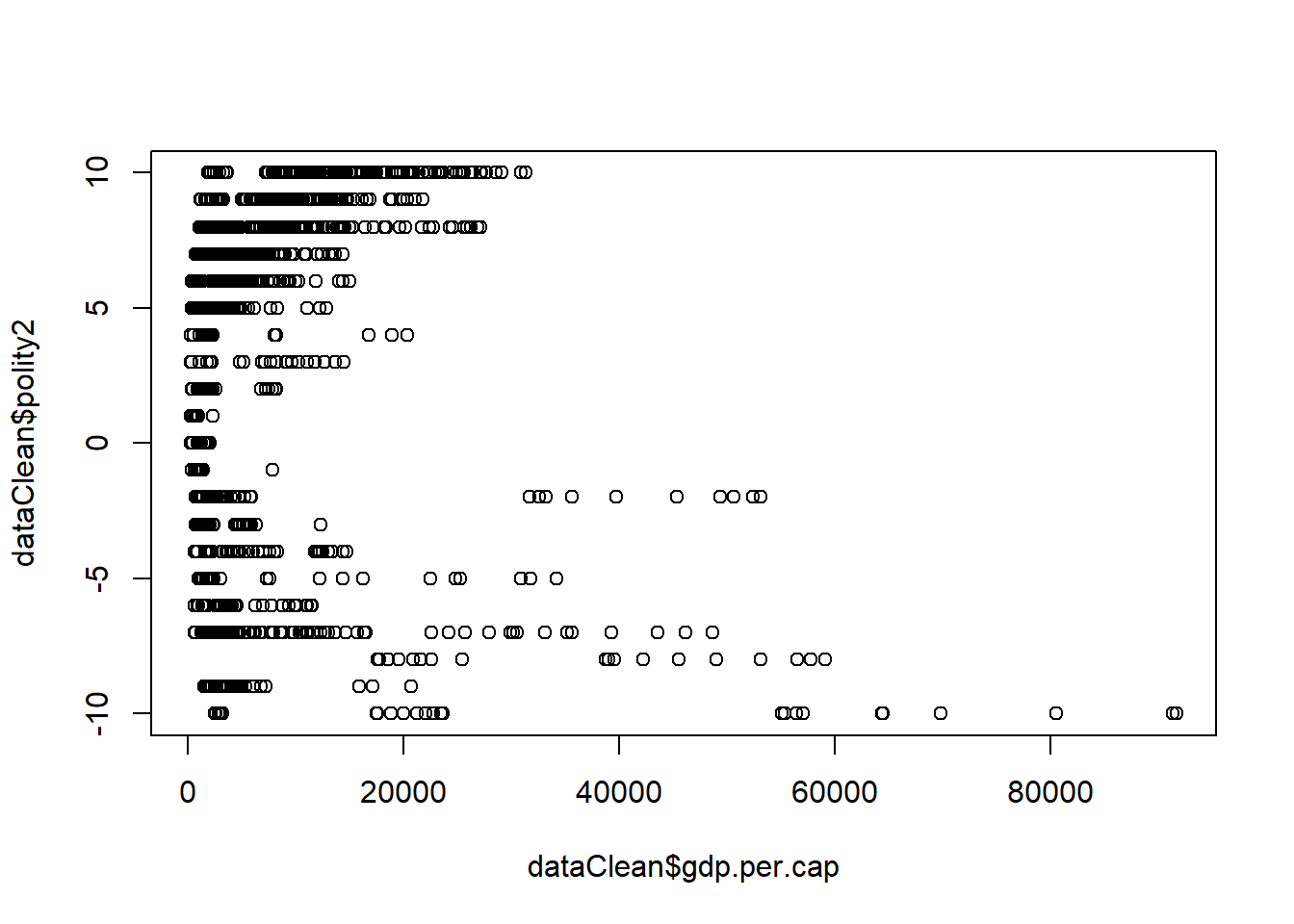# Try logging GDP
plot(x = log(dataClean$gdp.per.cap), y = dataClean$polity2,
xlab = "Logged GDP per capita",
ylab = "Polity2")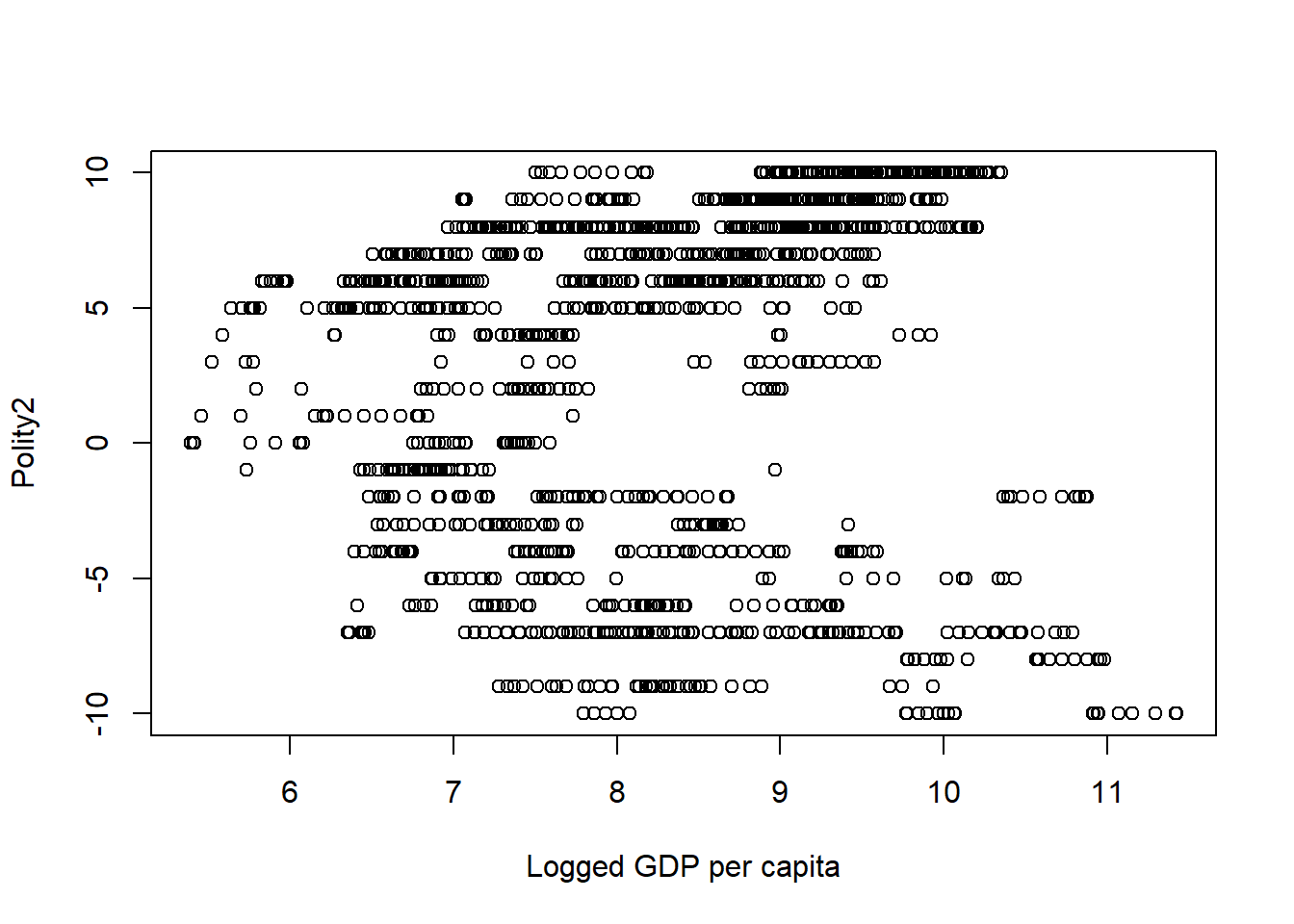# ggplot2
ggplot(dataClean, aes(y = polity2, x = log(gdp.per.cap))) +
geom_point() +
labs(x = "Logged GDP per capita", y = "Polity2") +
theme_classic()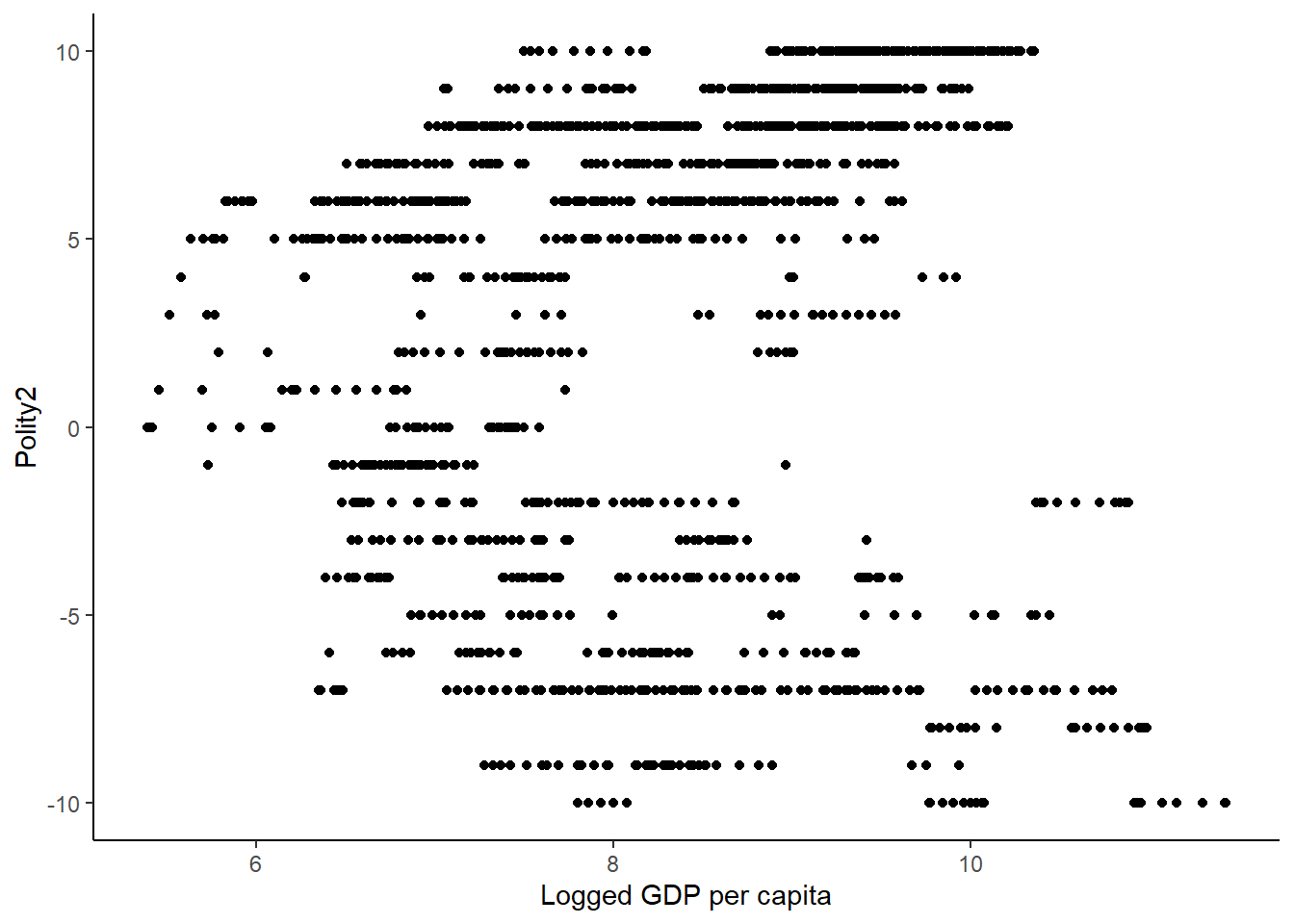## 7. Create a new variable called “democracy”. Assign 0 to countries with negative value or zero polity2 score, and assign 1 to countries with positive score.

# Create a variable called "democracy"
dataClean$democracy <- NA head(dataClean) # You can subset data based on a logical statement dataClean$polity2 <= 0

dataClean[dataClean$polity2 <= 0, ] # Take advantage of this: Assign values to "democracy" based on polity2 values dataClean$democracy[dataClean$polity2 <= 0] <- 0 # Do the same for positive Polity2 score dataClean$democracy[dataClean$polity2 > 0] <- 1 # Tidy way dataClean %>% mutate(democracy = case_when(polity2 <= 0 ~ 0, TRUE ~ 1)) ## 8. Use a loop to do the same coding. dataClean$democracy <- NA # clean this variable

n <- nrow(dataClean) # how many loops do you need?

for (i in 1:n) {

if (dataClean$polity2[i] <= 0) dataClean$democracy[i] <- 0

else dataClean$democracy[i] <- 1 } ## or try this for (i in 1:n) { dataClean$democracy <- ifelse(dataClean$polity2[i]<=0, 1, 0) } # Data Frames Practice 2 ## 1. Read in the data “lab1_survey.csv” # Clear and load data rm(list = ls()) survey_data <- read.csv(file = "lab1_survey.csv", encoding="UTF-8") # is this a good idea? ## 2. Inspect and view the data. What format are they in? What values do the data take, and how do those values correspond with the survey? View(survey_data) ## 2.1. How do you want to load properly and change the format into a plain data frame format? survey_data <- read.table(file = "lab1_survey.csv", sep=",", encoding="UTF-8") survey_data <- t(survey_data) # transpose items <- survey_data[1,] # save column names for items survey_data <- survey_data[-1,] # delete the name column n <- nrow(survey_data) # the number of total respondents id <- 1:n # id column rownames(survey_data) <- id colnames(survey_data) <- items survey_data <- as.data.frame(survey_data) # coerce all elements from character into numeric survey_data <- survey_data %>% mutate_all(function(x) as.numeric(as.character(x))) Need a shorter version? survey_data_2 <- read.table(file = "lab1_survey.csv", sep=",", encoding="UTF-8") %>% setNames(., c("item",1:19)) %>% gather(key=id, value=response, 2:20) %>% spread(key=item, value=response) %>% arrange(as.numeric(id)) %>% select(-id) #compare the results survey_data[13,] %>% sum() ##  76 survey_data_2[13,] %>% sum() ##  76 ## 3. Generate some summary statistics. colnames(survey_data) <- colnames(survey_data_2) <- "logit" summary(survey_data) ## logit probit ordered probit Bayes Theorem ## Min. :2.000 Min. :1.000 Min. :1.000 Min. :2.000 ## 1st Qu.:3.000 1st Qu.:2.000 1st Qu.:1.500 1st Qu.:2.000 ## Median :3.000 Median :3.000 Median :2.000 Median :2.000 ## Mean :3.158 Mean :2.526 Mean :2.105 Mean :2.684 ## 3rd Qu.:3.000 3rd Qu.:3.000 3rd Qu.:3.000 3rd Qu.:3.000 ## Max. :5.000 Max. :4.000 Max. :4.000 Max. :4.000 ## Maximum likelihood Negative Binomial Poisson distribution ## Min. :1.000 Min. :1.000 Min. :1.000 ## 1st Qu.:2.000 1st Qu.:2.000 1st Qu.:2.500 ## Median :2.000 Median :2.000 Median :3.000 ## Mean :2.211 Mean :2.211 Mean :2.842 ## 3rd Qu.:2.500 3rd Qu.:3.000 3rd Qu.:3.000 ## Max. :4.000 Max. :3.000 Max. :4.000 ## zero-inflated Poisson R LATEX stochastic simulation ## Min. :1.000 Min. :3.000 Min. :1.000 Min. :1.000 ## 1st Qu.:1.000 1st Qu.:3.000 1st Qu.:2.000 1st Qu.:1.000 ## Median :2.000 Median :3.000 Median :2.000 Median :1.000 ## Mean :1.842 Mean :3.474 Mean :2.421 Mean :1.526 ## 3rd Qu.:3.000 3rd Qu.:4.000 3rd Qu.:3.000 3rd Qu.:2.000 ## Max. :3.000 Max. :4.000 Max. :4.000 Max. :3.000 ## GLM random effects fixed effects selection bias ## Min. :1.000 Min. :2.000 Min. :2.000 Min. :2.000 ## 1st Qu.:2.500 1st Qu.:2.000 1st Qu.:2.000 1st Qu.:3.000 ## Median :3.000 Median :2.000 Median :3.000 Median :4.000 ## Mean :2.842 Mean :2.526 Mean :2.789 Mean :3.421 ## 3rd Qu.:3.000 3rd Qu.:3.000 3rd Qu.:3.000 3rd Qu.:4.000 ## Max. :4.000 Max. :4.000 Max. :4.000 Max. :4.000 ## prior distribution cross-validation tobit heteroskedasticity ## Min. :1.0 Min. :1.000 Min. :1.000 Min. :2.000 ## 1st Qu.:1.5 1st Qu.:1.000 1st Qu.:1.000 1st Qu.:3.000 ## Median :2.0 Median :2.000 Median :1.000 Median :3.000 ## Mean :2.0 Mean :1.947 Mean :1.316 Mean :3.105 ## 3rd Qu.:2.0 3rd Qu.:3.000 3rd Qu.:1.000 3rd Qu.:4.000 ## Max. :4.0 Max. :4.000 Max. :3.000 Max. :4.000 ## conditional logit hierarchical linear model gradient search bootstrap ## Min. :1.000 Min. :1.000 Min. :1.000 Min. :1.000 ## 1st Qu.:1.000 1st Qu.:2.000 1st Qu.:1.000 1st Qu.:2.000 ## Median :1.000 Median :2.000 Median :1.000 Median :2.000 ## Mean :1.421 Mean :1.947 Mean :1.263 Mean :2.474 ## 3rd Qu.:2.000 3rd Qu.:2.000 3rd Qu.:1.500 3rd Qu.:3.000 ## Max. :3.000 Max. :4.000 Max. :2.000 Max. :4.000 ## likelihood ratio AIC confidence interval first difference ## Min. :2.000 Min. :1.000 Min. :3.000 Min. :1.000 ## 1st Qu.:2.000 1st Qu.:2.000 1st Qu.:3.500 1st Qu.:1.000 ## Median :2.000 Median :3.000 Median :4.000 Median :1.000 ## Mean :2.579 Mean :2.526 Mean :3.737 Mean :1.316 ## 3rd Qu.:3.000 3rd Qu.:3.000 3rd Qu.:4.000 3rd Qu.:1.500 ## Max. :4.000 Max. :4.000 Max. :4.000 Max. :3.000 ## relative risk expected value multiple imputation ## Min. :1.000 Min. :2.000 Min. :1 ## 1st Qu.:1.500 1st Qu.:2.500 1st Qu.:2 ## Median :2.000 Median :3.000 Median :2 ## Mean :2.421 Mean :2.947 Mean :2 ## 3rd Qu.:3.000 3rd Qu.:3.000 3rd Qu.:2 ## Max. :4.000 Max. :4.000 Max. :4 mean(survey_data$R)
##  3.473684
mean(survey_data$LATEX) ##  2.421053 median(survey_data$R)
##  3
median(survey_data$LATEX) ##  2 sd(survey_data$R)
##  0.5129892
sd(survey_data\$LATEX)
##  0.7685332
# Tidy way
survey_data %>%
summarize_all(funs(mean, median, sd, min, max)) %>%
gather(key = "stat")
## Warning: funs() was deprecated in dplyr 0.8.0.
## Please use a list of either functions or lambdas:
##
##   # Simple named list:
##   list(mean = mean, median = median)
##
##   # Auto named with tibble::lst():
##   tibble::lst(mean, median)
##
##   # Using lambdas
##   list(~ mean(., trim = .2), ~ median(., na.rm = TRUE))
# Which item has the highest and lowest mean value?
survey_data %>%
summarize_all(funs(mean)) %>%
gather(key = "stat") %>%
filter(rank(value)==1 | rank(value)==30)

## 6. Recode the data

survey_data %>%
mutate(# Recode R into categories
R_cat = case_when(R == 1 ~ "What's that?",
R == 2 ~ "I've heard of it",
R == 3 ~ "I can use it or apply it",
TRUE ~ "I understand it well"),
# Recode latex into categories
LATEX_cat = case_when(LATEX == 1 ~ "What's that?",
LATEX == 2 ~ "I've heard of it",
LATEX == 3 ~ "I can use it or apply it",
TRUE ~ "I understand it well"))
# We're repeating ourselves... Must be a faster way
survey_data <-
survey_data %>%
mutate_at(vars(R, LATEX),
function(x) case_when(x == 1 ~ "What's that?",
x == 2 ~ "I've heard of it",
x == 3 ~ "I can use it or apply it",
TRUE ~ "I understand it well"))

## 8. Generate some plots of the data: bar charts are good here, scatterplots even better.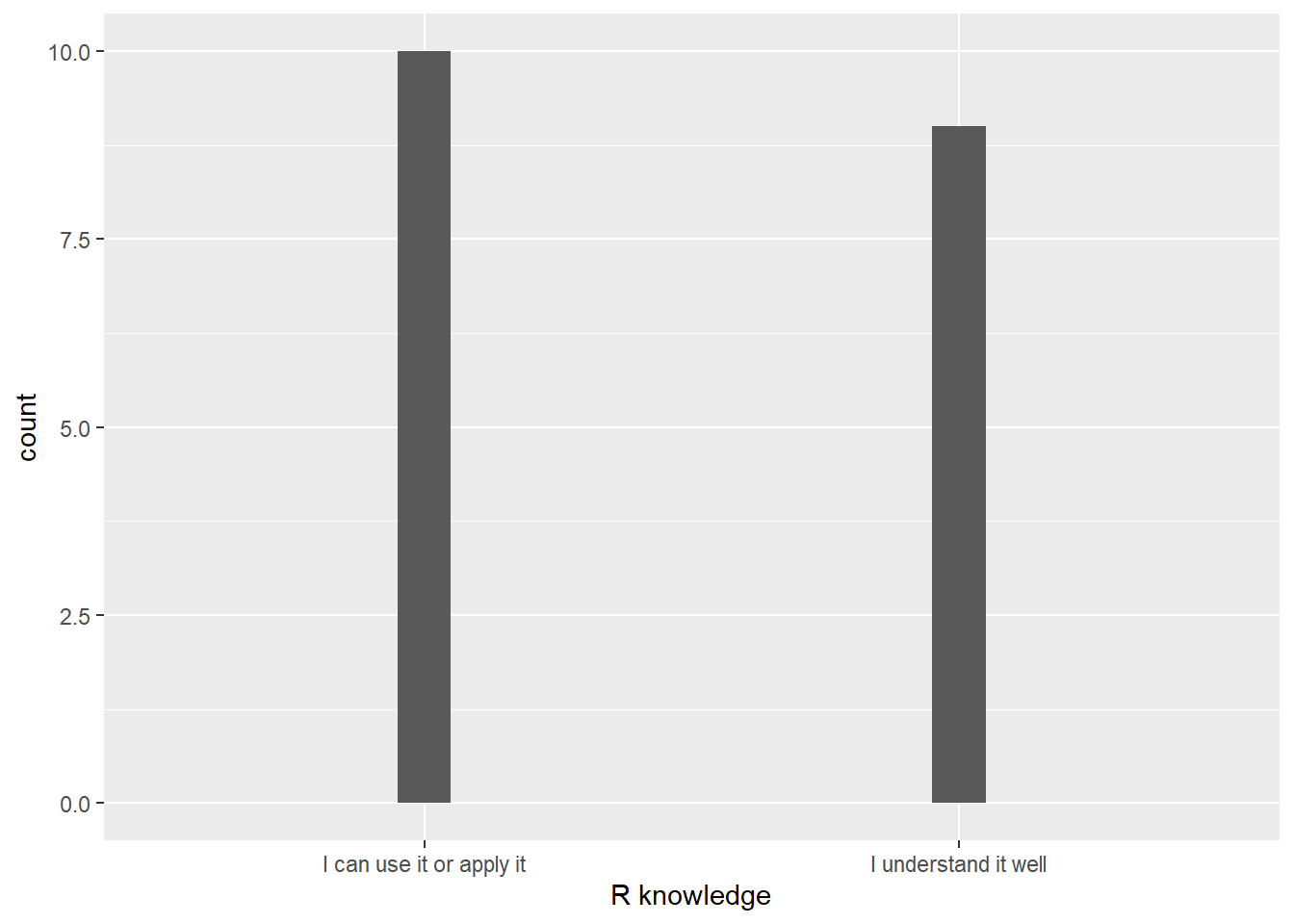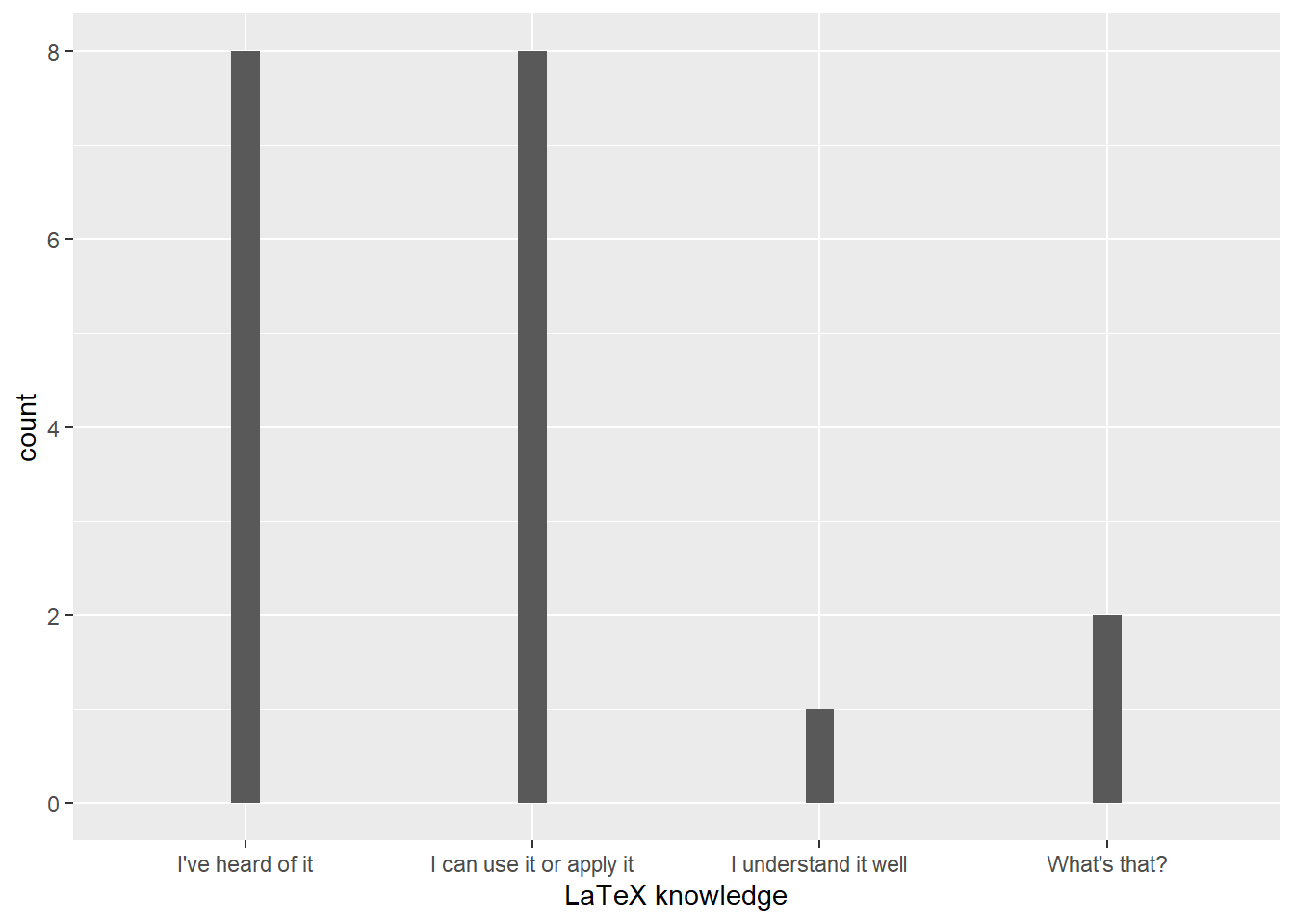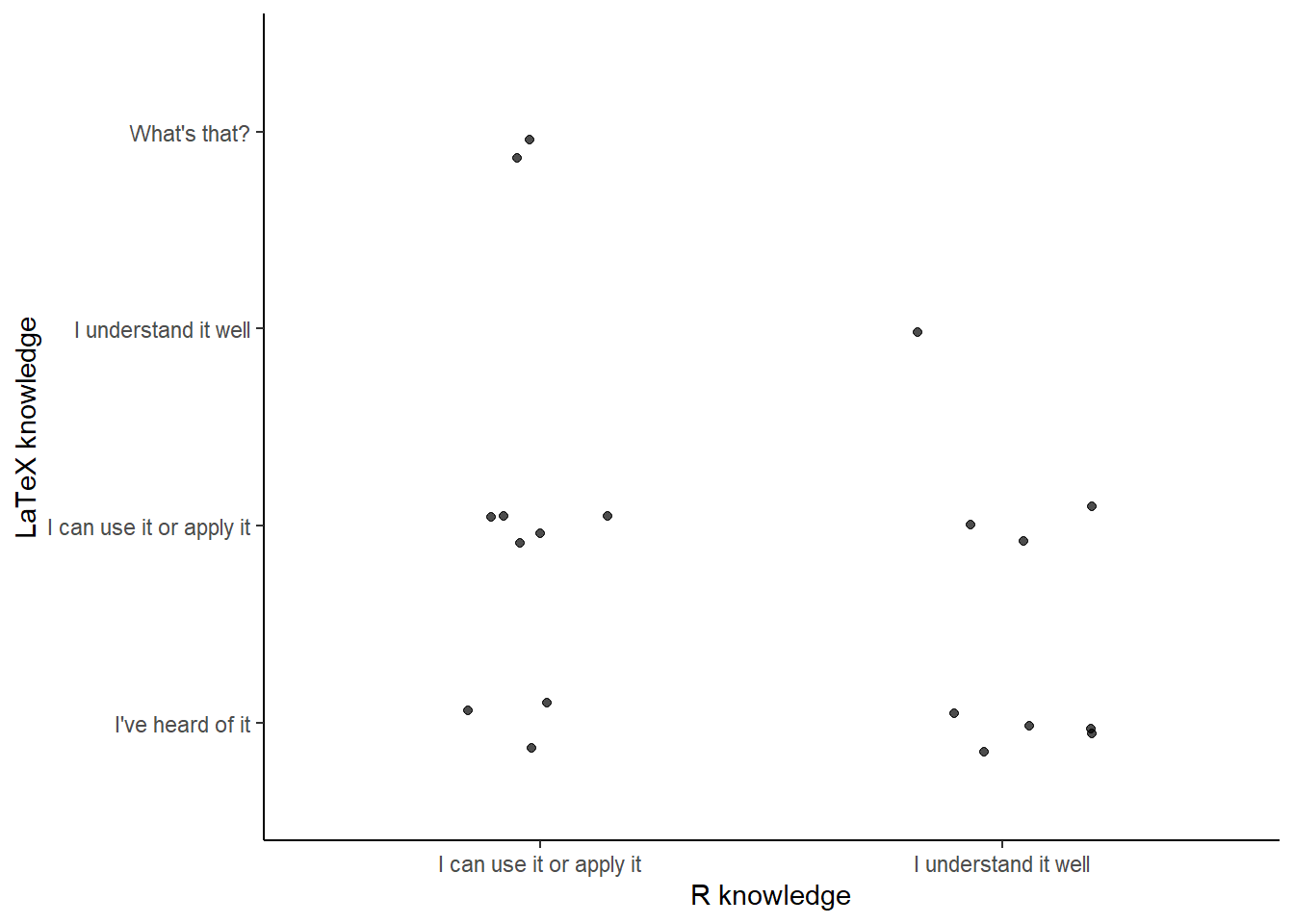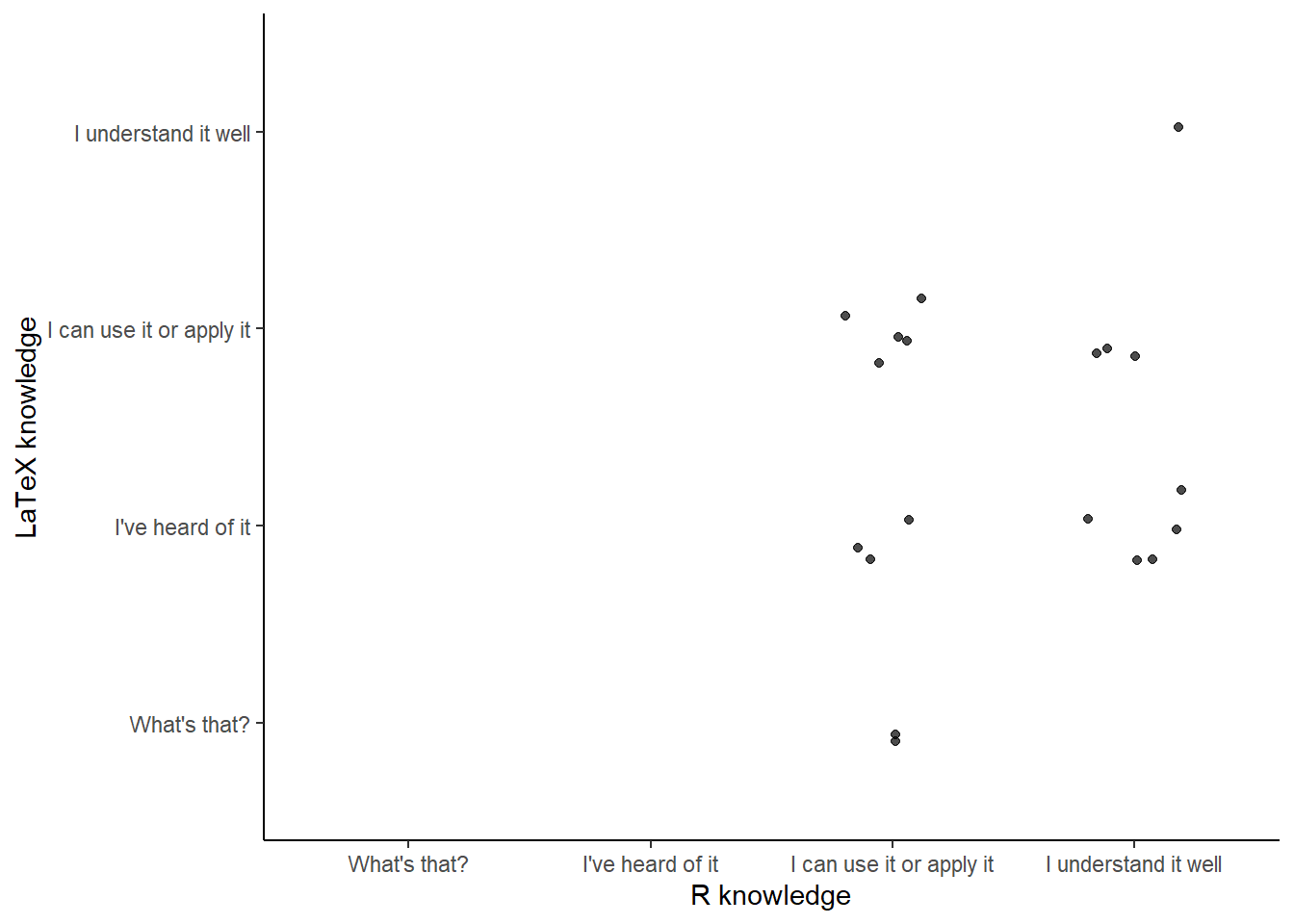# LaTex in R Markdown

$1 + 1 = 2$

$11 \times 11 = 121 \\$

$E = mc^2$

I think it’s Einstein who proposed $$E = mc^2$$.

$x = \frac{-b \pm \sqrt{b^2 - 4ac}}{2a}$

$\begin{split} X & = (x+a)(x-b) \\ & = x(x-b) + a(x-b) \\ & = x^2 + x(a-b) - ab \end{split}$ Chris recommends using LaTeX functions rather than dollar signs, such as:

$\begin{equation} x = \frac{-b \pm \sqrt{b^2 - 4ac}}{2a} \end{equation}$

$\begin{equation} \textrm{response}_i = \alpha_i + \beta \mathrm{covariate}_i + \varepsilon_i \end{equation}$

$\begin{eqnarray} \textrm{response}_i & = & \alpha_i \\ && + \beta \mathrm{covariate}_i + \varepsilon_i \end{eqnarray}$